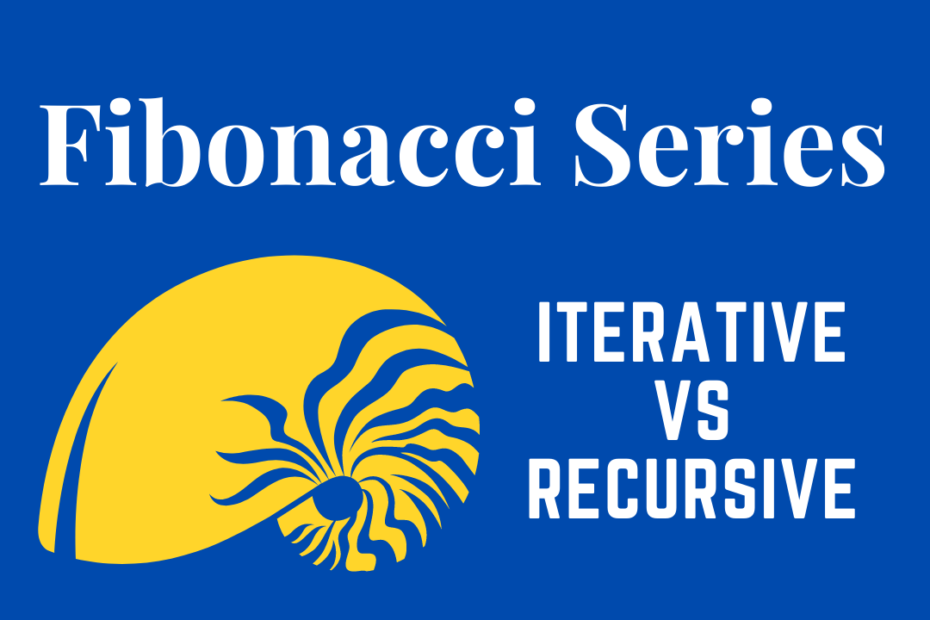# Fibonacci Series – Iterative vs Recursive

• DSA

The Fibonacci Series is a standard programming problem scenario, and we can obtain the series or nth Fibonacci number using both iterative as well as recursive. In this post, we’ll compare, discuss both methods and their complexities.

## Fibonacci Series – Example

``0 1 1 2 3 5 8 13 21 34 55 89 144``

The principle behind this order/sequence is very simple

The third number will be the sum of first two numbers and this keep repeating

The Mathematical formula would be, Fn = Fn-1 + Fn-2

``````0 1
0 1 1 (0+1)
0 1 1 2 (1+2)
0 1 1 2 3 (2+3)``````

## Fibonacci – Iterative Method

``````#include <iostream>

int Fibonacci(int n)
{
int i, one = 0, two = 1, three;
if (n == 0)
return one;
for (i = 2; i <= n; i++)
{
three = one + two;
one = two;
two = three;
}
return two;
}

int main()
{
int n = 10;
std::cout<<Fibonacci(n);
return 0;
}``````

Time Complexity: O(N)

Space Complexity: O(1)

## Explanation

Here we iterate n no.of times to find the nth Fibonacci number nothing more or less, hence time complexity is O(N), and space is constant as we use only three variables to store the last 2 Fibonacci numbers to find the next and so on.

## Fibonacci Series- Recursive Method C++

``````#include <iostream>

int Fibonacci(int n)
{
if (n <= 1)
return n;
return Fibonacci(n-1) + Fibonacci(n-2);
}

int main ()
{
int n = 3;
std::cout << Fibonacci(n);
return 0;
}``````

Time Complexity: O(2N) Exponential

Space Complexity: O(N)

## Explanation

First, we’ll consider the Time Complexity, for example

If n > 1 then T(n) = T(n-1) + T(n-2), because each recursion would call two more making the Time Complexity Exponential

Space looks constant but every time recursion is carried out there is a lot going on in the background as stack memory is used up for every call.

## Which one is the Fastest?

It’s obvious, Iterative method is the fastest as well as memory efficient as we store only the last two values.

This post part of my #30DaysChallenge to write a blog post every day.